It can be frustrating when the same probability distribution has two different parameterizations, but such is the life of a statistical programmer. I previously wrote an article about the gamma distribution, which has two common parameterizations: one that uses a scale parameter (β) and another that uses a rate parameter (c = 1/β).

The relationship between scale and rate parameters is straightforward, but sometimes the relationship between different parameterizations is more complicated. Recently, a SAS programmer was using a regression procedure to fit the parameters of a Weibull distribution. He was confused about how the output from a SAS regression procedure relates to a more familiar parameterization of the Weibull distribution, such as is fit by PROC UNIVARIATE. This article shows how to perform two-parameter Weibull regression in several SAS procedures, including PROC RELIABILITY, PROC LIFEREG, and PROC FMM. The parameter estimates from regression procedures are not the usual Weibull parameters, but you can transform them into the Weibull parameters.

This article fits a two-parameter Weibull model. In a two-parameter model, the threshold parameter is assumed to be 0. A zero threshold assumes that the data can be any positive value.

### Fitting a Weibull distribution in PROC UNIVARIATE

PROC UNIVARIATE is the first tool to reach for if you want to fit a Weibull distribution in SAS. The most common parameterization of the Weibull density is
$f(x; \alpha, \beta) = \frac{\beta}{\alpha^{\beta}} (x)^{\beta -1} \exp \left(-\left(\frac{x}{\alpha}\right)^{\beta }\right)$
where α is a shape parameter and β is a scale parameter. This parameterization is used by most Base SAS functions and procedures, as well as many regression procedures in SAS. The following SAS DATA step simulates data from the Weibull(α=1.5, β=0.8) distribution and fits the parameters by using PROC UNIVARIATE:

/* sample from a Weibull distribution */ %let N = 100; data Have(drop=i); call streaminit(12345); do i = 1 to &N; d = rand("Weibull", 1.5, 0.8); /* Shape=1.5; Scale=0.8 */ output; end; run;   proc univariate data=Have; var d; histogram d / weibull endpoints=(0 to 2.5 by 0.25); /* fit Weib(Sigma, C) to the data */ probplot / weibull2(C=1.383539 SCALE=0.684287) grid; /* OPTIONAL: P-P plot */ ods select Histogram ParameterEstimates ProbPlot; run;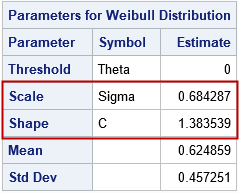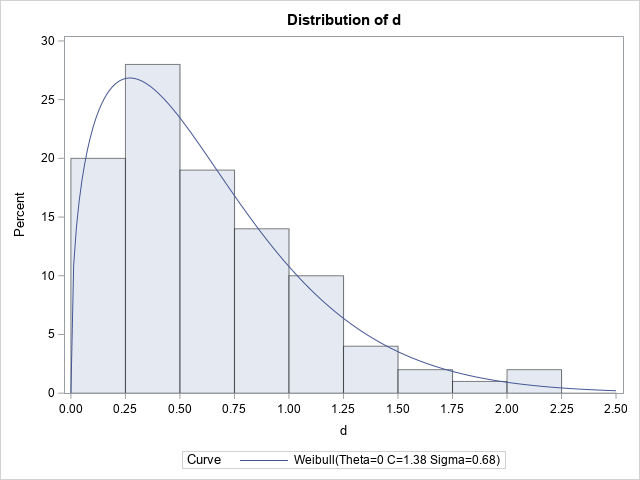The histogram of the simulated data is overlaid with a density from the fitted Weibull distribution. The parameter estimates are Shape=1.38 and Scale=0.68, which are close to the parameter values. PROC UNIVARIATE uses the symbols c and σ for the shape and scale parameters, respectively.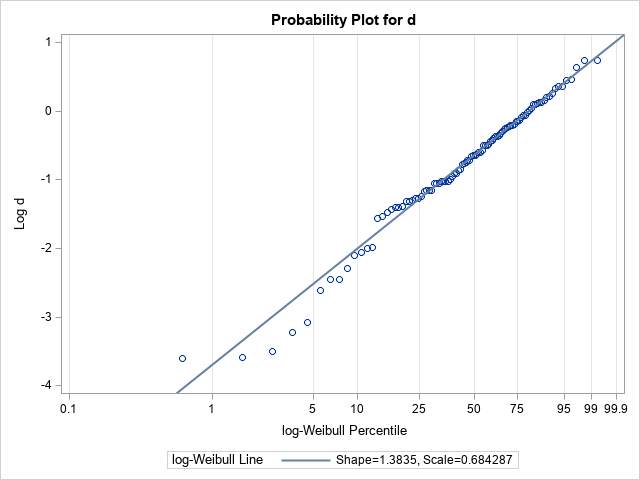The probability-probability (P-P) plot for the Weibull distribution is shown. In the P-P plot, a reference line is added by using the option weibull2(C=1.383539 SCALE=0.684287). (In practice, you must run the procedure once to get those estimates, then a second time to plot the P-P plot.) The slope of the reference line is 1/Shape = 0.72 and the intercept of the reference line is log(Scale) = -0.38. Notice that the P-P plot is plotting the quantiles of log(d), not of d itself.

### Weibull regression versus univariate fit

It might seem strange to use a regression procedure to fit a univariate distribution, but as I have explained before, there are many situations for which a regression procedure is a good choice for performing a univariate analysis. Several SAS regression parameters can fit Weibull models. In these models, it is usually assumed that the response variable is a time until some event happens (such as failure, death, or occurrence of a disease). The documentation for PROC LIFEREG provides an overview of fitting a model where the logarithm of the random errors follows a Weibull distribution. In this article, we do not use any covariates. We simply model the mean and scale of the response variable.

A problem with using a regression procedure is that a regression model provides estimates for intercepts, slopes, and scales. It is not always intuitive to see how those regression estimates relate to the more familiar parameters for the probability distribution. However, the P-P plot in the previous section shows how intercepts and slopes can be related to parameters of a distribution. The documentation for the LIFEREG procedure states that the Weibull scale parameter is exp(Intercept) and the Weibull shape parameter is the reciprocal of the regression scale parameter.

Notice how confusing this is! For the Weibull distribution, the regression model estimates a SCALE parameter for the error distribution. But the reciprocal of that scale estimate is the Weibull SHAPE parameter, NOT the Weibull scale parameter! (In this article, the response distribution and the error distribution are the same.)

### Weibull regression in SAS

The LIFEREG procedure includes an option to produce a probability-probability (P-P) plot, which is similar to a Q-Q plot. The LIFEREG procedure also estimates not only the regression parameters but also provides estimates for the exp(Intercept) and 1/Scale quantities. The following statements use a Weibull regression model to fit the simulated data:

title "Weibull Estimates from LIFEREG Procedure"; proc lifereg data=Have; model d = / dist=Weibull; probplot; inset; run;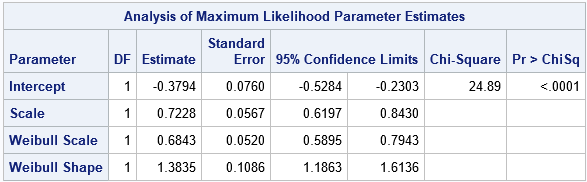The ParameterEstimates table shows the estimates for the Intercept (-0.38) and Scale (0.72) parameters in the Weibull regression model. We previously saw these numbers as the parameters of the reference line in the P-P plot from PROC UNIVARIATE. Here, they are the result of a maximum likelihood estimate for the regression model. To get from these values to the Weibull parameter estimates, you need to compute Weib_Scale = exp(Intercept) = 0.68 and Weib_Shape = 1/Scale = 1.38. PROC LIFEREG estimates these quantities for you and provides standard errors and confidence intervals.

The graphical output of the PROBPLOT statement is equivalent to the P-P plot in PROC UNIVARIATE, except that PROC LIFEREG reverses the axes and automatically adds the reference line and a confidence band.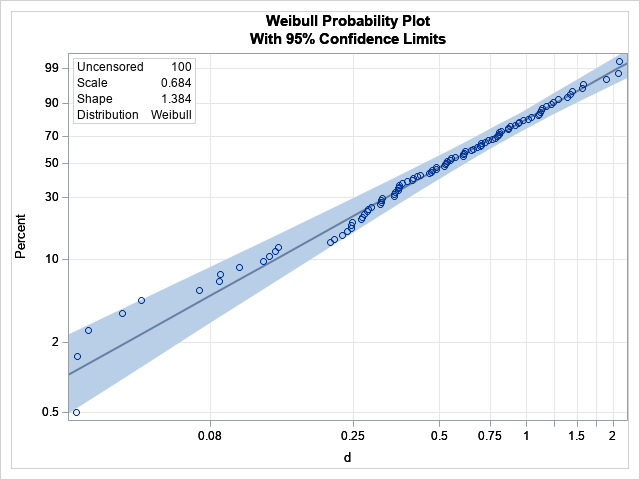### Other regression procedures

Before ending this article, I want to mention two other regression procedures that perform similar computations: PROC RELIABILITY, which is in SAS/QC software, and PROC FMM in SAS/STAT software.

The following statements call PROC RELIABILITY to fit a regression model to the simulated data:

title "Weibull Estimates from RELIABILITY Procedure"; proc reliability data=Have; distribution Weibull; model d = ; run;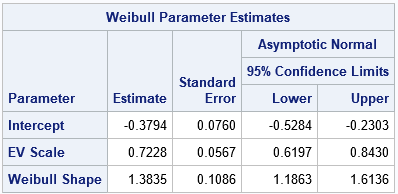The parameter estimates are similar to the estimates from PROC LIFEREG. The output also includes an estimate of the Weibull shape parameter, which is 1/EV_Scale. The output does not include an estimate for the Weibull scale parameter, which is exp(Intercept).

In a similar way, you can use PROC FMM to fit a Weibull model. PROC FMM Is typically used to fix a mixture distribution, but you can specify the K=1 option to fit a single response distribution, as follows:

title "Weibull Estimates from FMM Procedure"; proc fmm data=Have; model d = / dist=weibull link=log k=1; ods select ParameterEstimates; run;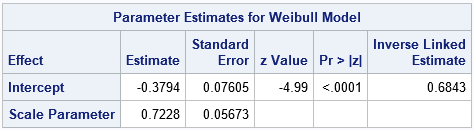The ParameterEstimates table shows the estimates for the Intercept (-0.38) and Scale (0.72) parameters in the Weibull regression model. The Weibull scale parameter is shown in the column labeled "Inverse Linked Estimate." (The model uses a LOG link, so the inverse link is EXP.) There is no estimate for the Weibull shape parameter. which is the reciprocal of the Scale estimate.

### Summary

The easiest way to fit a Weibull distribution to univariate data is to use the UNIVARIATE procedure in Base SAS. The Weibull shape and scale parameters are directly estimated by that procedure. However, you can also fit a Weibull model by using a SAS regression procedure. If you do this, the regression parameters are the Intercept and the scale of the error distribution. You can transform these estimates into estimates for the Weibull shape and scale parameters. This article shows the output (and how to interpret it) for several SAS procedures that can fit a Weibull regression model.

Why would you want to use a regression procedure instead of PROC UNIVARIATE? One reason is that the response variable (failure or survival) might depend on additional covariates. A regression model enables you to account for additional covariates and still understand the underlying distribution of the random errors. A second reason is that the FMM procedure can fit a mixture of distributions. To make sense of the results, you must be able to interpret the regression output in terms of the usual parameters for the probability distributions.

The post Interpret estimates for a Weibull regression model in SAS appeared first on The DO Loop.